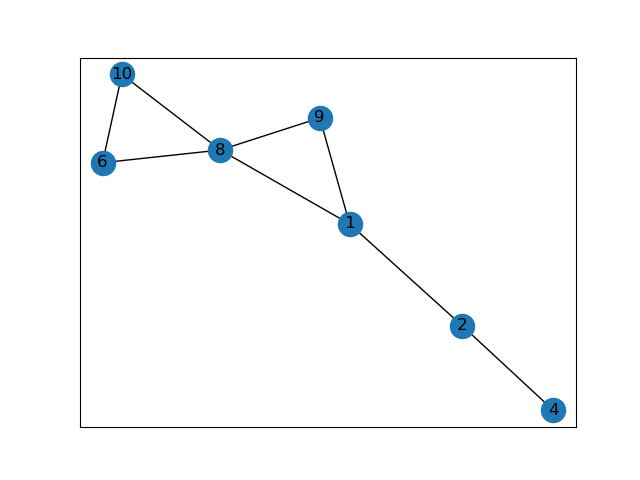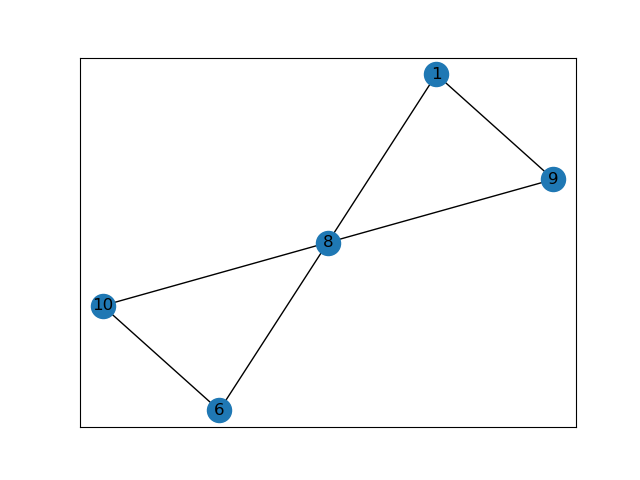# [PYTHON] Delete vertices that meet the conditions in networkx

Let's delete the vertices that meet the conditions from the graph defined in the program!

### This condition

--Use networkx --Graphs are defined in the program -Delete [Vertex with degree 0] or [Vertex with degree 1 and the edge connected to that vertex].

# The first program I thought of

``````import networkx as nx
import matplotlib.pyplot as plt
G=nx.Graph()

#n=G.number_of_nodes()
#[Vertices of degree 0]Or[Vertices of degree 1 and edges connecting to those vertices]To delete
for v in G:
#print(G.degree(v))
#print("v=",v)
G_deg=G.degree(v)
if G_deg==0 or G_deg==1:
G.remove_node(v)
#print("G_deg=",G_deg)

#Graph output
nx.draw_networkx(G)
plt.show()
``````

I thought it was good and tried it.

### error

``````Traceback (most recent call last):
File "kadai06d.py", line 10, in <module>
for v in G:
RuntimeError: dictionary changed size during iteration
``````

It's not straightforward. .. .. I searched online to find the cause.

### Cause 1

First of all, the meaning of the error is ** "The iterating object cannot be changed" **. In other words, does it mean that you can't delete while looping with `for v in G:`?

# Improvement 1

If I couldn't delete it inside `for v in G`, I thought I would list the vertices that meet the conditions and then delete them all at once outside` for v in G`.

### Improvement 1 program

``````import networkx as nx
import matplotlib.pyplot as plt
G=nx.Graph()

node_be=G.number_of_nodes() #Number of vertices before deletion
edge_be=G.number_of_edges() #Number of sides before deletion

#[Vertices of degree 0]Or[Vertices of degree 1 and edges connecting to those vertices]To delete
G_rem=[]
#List the vertices that meet the conditions
for v in G:
G_deg=G.degree(v)
#print("G_deg[",v,"]=",G_deg)
if G_deg<=1:
#print("v=",v)
G_rem.append(v)

#Delete vertices that meet the conditions
G.remove_nodes_from(G_rem)

node_af=G.number_of_nodes() #Number of vertices after deletion
edge_af=G.number_of_edges() #Number of edges after deletion

#Graph output
nx.draw_networkx(G)
plt.show()
``````

I tried to run it with this.

#### Executed graph\\ There are still vertices of degree 1 ///

### Cause 2

Just by looking at `for v in G:` once, it seems that `v` is getting bigger and bigger and even if the order becomes 1, it goes through. In other words, you have to do it once and look back over and over again!

# Improvement 2

I decided to do a double loop to repeat `for v in G:` many times. Try turning `for` many times for the number of vertices.

### Improved program

``````import networkx as nx
import matplotlib.pyplot as plt
G=nx.Graph()

node_be=G.number_of_nodes() #Number of vertices before deletion
edge_be=G.number_of_edges() #Number of sides before deletion

#[Vertices of degree 0]Or[Vertices of degree 1 and edges connecting to those vertices]To delete
G_rem=[]
#List the vertices that meet the conditions
for i in range(node_be):
for v in G:
G_deg=G.degree(v)
#print("G_deg[",v,"]=",G_deg)
if G_deg<=1:
#print("v=",v)
G_rem.append(v)
#Delete vertices that meet the conditions
G.remove_nodes_from(G_rem)

node_af=G.number_of_nodes() #Number of vertices after deletion
edge_af=G.number_of_edges() #Number of edges after deletion

#Graph output
nx.draw_networkx(G)
plt.savefig("output.png ")
plt.show()
``````

#### Executed graphThe apex of degree 1 is gone!

# Finally

I found that I couldn't easily delete vertices without going through various steps. Thank you until the end.